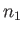Next: Exercises Up: Probability Theory Previous: Gaussian Probability Distribution

# Central Limit Theorem

It may appear, at first sight, that the Gaussian distribution function is only relevant to two-state systems. In fact, as we shall see, the Gaussian probability distribution is of crucial importance to statistical physics because, under certain circumstances, it applies to all types of system.

Let us briefly review how we obtained the Gaussian distribution function in the first place. We started from a very simple system with only two possible outcomes. Of course, the probability distribution function (for) for this system did not look anything like a Gaussian. However, when we combined very many of these simple systems together, to produce a complicated system with a great number of possible outcomes, we found that the resultant probability distribution function (for) reduced to a Gaussian in the limit that the number of simple systems tended to infinity. We started from a two outcome system because it was easy to calculate the final probability distribution function when a finite number of such systems were combined together. Clearly, if we had started from a more complicated system then this calculation would have been far more difficult.

Suppose that we start from a general system, with a general probability distribution function (for some measurable quantity). It turns out that if we combine a sufficiently large number of such systems together then the resultant distribution function (for) is always Gaussian. This astonishing result is known as the central limit theorem. Unfortunately, the central limit theorem is notoriously difficult to prove. A somewhat restricted proof is presented in Sections 1.10 and 1.11 of Reif. The central limit theorem guarantees that the probability distribution of any measurable quantity is Gaussian, provided that a sufficiently large number of statistically independent observations are made. We can, therefore, confidently predict that Gaussian probability distributions are going to crop up very frequently in statistical thermodynamics.Next: Exercises Up: Probability Theory Previous: Gaussian Probability Distribution
Richard Fitzpatrick 2016-01-25